The energy measurement chip used in the WNB and WNC series WattNode® meters does not directly report the line-to-line (or phase-to-phase) voltage. These voltages can be estimated mathematically by the WattNode BACnet and Modbus meters by selecting the PhaseOffset parameter corresponding to type of electrical service being measured. Refer to the PhaseOffset section in the WattNode BACnet Manual or the WattNode Modbus manual for details.

For firmware versions 15 and lower, WattNode Modbus only estimates line-to-line voltages for 120/240 Vac residential services and for standard three-phase circuits, not including four wire delta circuits (aka “wild leg” or “high phase”), and not including grounded delta circuits. Firmware versions 16 and later add estimated line-to-line voltages for four-wire delta and grounded delta circuits. See the PhaseOffset register in the WattNode Modbus manual for details.

## Standard Three-Phase Circuits

The following equations should provide accurate estimated line-to-line voltages provided the three phases are separated by 120° and the neutral (or ground if neutral is absent) to line voltages are well balanced (within a few percent).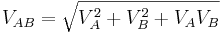$V_{AB} = \sqrt{V_A^2 + V_B^2 + V_A V_B}$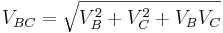$V_{BC} = \sqrt{V_B^2 + V_C^2 + V_B V_C}$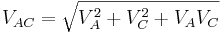$V_{AC} = \sqrt{V_A^2 + V_C^2 + V_A V_C}$

## Residential Circuits

North American residential circuits typically use two 120 Vac lines that are 180° apart, with a line-to-line voltage of 240 Vac. Because of the 180° angle between the two lines, the individual voltages can be added to accurately estimate the line-to-line voltage.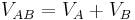$V_{AB} = V_A + V_B$

## Three-Phase Grounded Delta Circuits

Three-phase three-wire grounded delta circuits (also called “corner-grounded delta” or “grounded leg”) have one of the phases tied to ground.

Assuming phase B is the grounded leg, the following equations compute the line-to-line voltages. If a different phase is grounded, the equations can be shifted up or down a phase to get the correct answer: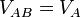$V_{AB} = V_A$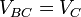$V_{BC} = V_C$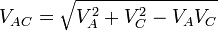$V_{AC} = \sqrt{V_A^2 + V_C^2 - V_A V_C}$

## Four-Wire Delta Circuits

Four-wire delta circuits (also called “wild leg” or “high leg”) have one phase with a higher line-to-neutral voltage than the other two. Most commonly, the line-to-neutral voltages are 120, 120, and 208 Vac, while the line-to-line voltages are all 240 Vac.

Assuming phase B is the high (or wild) leg, the following equations compute the line-to-line voltages. If a different phase is the high leg, the equations can be shifted up or down a phase to get the correct answer: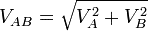$V_{AB} = \sqrt{V_A^2 + V_B^2}$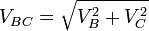$V_{BC} = \sqrt{V_B^2 + V_C^2}$$V_{AC} = V_A + V_C$

Keywords: phase offset, line to line, phase-to-phase, line-to-line